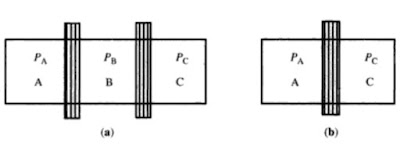# Laws of Thermodynamics | Zeroth Law, 1st Law, 2nd Law, 3rd Law of Thermodynamics

Zeroth Law of Thermodynamics, The first Law, Second Law and Third Law of Thermodynamics. Kelvin-Planck statement, Clausius statement of the second law

## Zeroth Law of Thermodynamics

According to the zeroth law of thermodynamics, "if two systems A and B are in thermal equilibrium with a third system C, then they must be in thermal equilibrium with each other."Three system A,B and C which are in thermal contact

This above statement is called the zeroth law of thermodynamics and forms the basis of concept of temperature. All these three systems can be said to process a property that ensures their being in thermal equilibrium with one another. This property is known as temperatures. We may, therefore, define the temperature of a system as the property that determines whether or not the system is in thermal equilibrium with the other neighbouring systems. It is clear that if two systems are not in thermal equilibrium, they will be at different temperatures.

## The first Law of Thermodynamics

The first law of thermodynamics is a particular form of the general law of conservation of energy. Suppose the amount of heat Q is supplied to a system. It is normally spent in two ways.

1. Partially, it is spent in increasing internal energy of system.
2. The remaining part of it is spent in expanding the body against the external pressure, i.e. in doing external work W.

If ΔU the change in internal energy "since energy can neither be created nor destroyed but only convert from one form to another", we have then

Q = ΔU + W............(1)

If dQ, dU and dW are infinitesimal changes in heat, internal energy and work respectively, then equation (1) becomes

dQ = dU + dW

This equation represents the differential form of first law of thermodynamics, which may therefore be stated. "In all transformations, the energy due to the supplied heat units must be balanced by an increase in the external work as well as the internal energy".

### Limitations of First Law of Thermodynamics

The first law of thermodynamics is basically the principle of conservation of energy. It plays an important role in thermodynamics as it can be applied to know how much work will be obtained by transferring a certain amount of heat energy in a given thermodynamic process. However, first law of thermodynamics suffers from the following limitations :

• First law of thermodynamics does not indicate the direction of heat transfer.
• First law of thermodynamics does not tell anything about the conditions under which heat can be transformed into work.
• The first law does not indicate as to why the whole of the heat energy cannot be continuously converted into mechanical work.

## Second Law of Thermodynamics

This has two statements. First is Kelvin-Planck statement which is based upon the performance of heat engine and second is Clausius statement which is based upon the performance of refrigerator.

### Kelvin-Planck statement of the second law

This may be stated as, "It is impossible to construct a device which operating in a cycle, has a sole effect of extracting heat from a reservoir at performing an equivalent amount of work". Thus, a single reservoir at a single temperature can not continuously transfer heat into work.

### Clausius statement of the second law

This may be stated as, "It is impossible for a self-acting machines working in a cycle process, unaided by any external agency to transfer heat from a body at a lower temperature to a body at a higher temperature." In other words it may be stated as "Heat cannot flow itself from a colder to a hotter body".

This is statement is based upon the performance of refrigerator-a heat engine working in the backward direction. In a refrigerator the working substance takes in  heat from a cold body, a certain amount of work is done on it by some outside agency and rejects a larger amount of heat to a hotter body. Thus a refrigerator transfers heat from a cold body to a hot body with the aid of an outside agency. No refrigerator has every been constructed which can do this work without the supply of energy from an external agency.

## Third Law of Thermodynamics

According to this law, "At absolute zero temperature, the entropy tends to zero and the molecules of a substance or a systems are in perfect order (well arranged). This is the third law of thermodynamics".

In this universe the entropy is increasing and ultimately the universe will also reached a maximum value of entropy when no work will be possible. With the increase in entropy, the disorder of the molecules of a substance increases. The entropy is also measured the disorder of the system. With decrease in entropy, the disorder decreases.